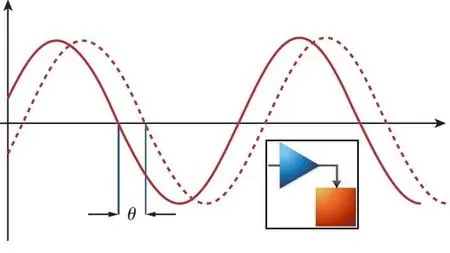AC Circuit & Power Analysis using MATLAB SIMULINKThanks:  0Likes:  0Dislikes:  0

# Tema: AC Circuit & Power Analysis using MATLAB SIMULINK

1. ##AC Circuit & Power Analysis using MATLAB SIMULINKAC Circuit & Power Analysis using MATLAB SIMU
.MP4 | Video: 1280x720, 30 fps(r) | Audio: AAC, 44100 Hz, 2ch | 2.12 GB
Duration: 4 hours | Genre: eLearning | Language: English

Have fun with designing your AC circuits and computing all required parameters

What you'll learn

Revise the basics of AC power analysis.

Measure different parameters in the AC circuits.

You should be familiar with basics of AC circuits.

What you'll learn
Revise the basics of AC circuit analysis.
Revise the basics of AC power analysis.
Learn how to design AC circuits using MATLAB SIMU.
Measure different parameters in the AC circuits.
Requirements
You should be familiar with basics of AC circuits.
Description
Circuit analysis, or solving a circuit, means figuring out voltages and currents in each element. This course starts off covering how to design your AC circuits using MATLAB SIMU and compute all required parameters including but not limited to the following:
Voltage.
Current.
Impedance.
Active Power.
Reactive Power.
Apparent Power.
Power Factor.
This course will help you to revise the basics of AC circuit and power analysis and to be skilled in using MATLAB SIMU.
Who this course is for:
Electrical Engineering Students.

rapidgator

nitroflare2.

####Permisos de publicación

• No puedes crear nuevos temas
• No puedes responder temas
• No puedes subir archivos adjuntos
• No puedes editar tus mensajes
•